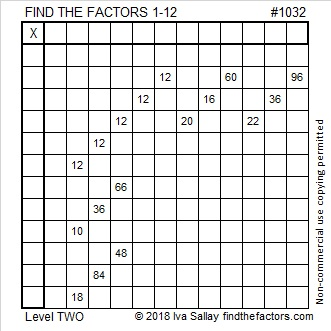# 1032 How Many Twelves Are in a 12×12 Times Table?

How many 12’s are in a standard 12×12 times table? There are five 12’s in the puzzle below. Six, if you count the 12 in the title. Is that too many, just the right number, or are there even more?

This is only a level 2 puzzle so it won’t be difficult to solve. . . unless I’ve put in too many 12’s!

Hmm. . .Try solving the puzzle, then fill in the rest of the multiplication table. Then you will know for sure how many 12’s SHOULD be in the table.Print the puzzles or type the solution in this excel file: 12 factors 1028-1034

The number 1032 is divisible by 12. Here are a few more facts about that number:

1032 is made with a zero and three consecutive numbers so it is divisible by 3.

The last two digits of 1032 are 32 so 1032 can be evenly divided by 4.

Since 32 is divisible by 8 and preceded by an even zero in 1032, our number is also divisible by 8.

As you will soon see, 1032 is divisible by even more numbers than those listed above. Here are three of its factor trees:1032 looks interesting in a couple of different bases:
It’s 4440 in BASE 6 because 4(6³ + 6² + 6¹) = 4(258) = 1032, and
it’s palindrome 3003 in BASE 7 because 3(7³ + 7⁰) = 3(344) = 1032.

• 1032 is a composite number.
• Prime factorization: 1032 = 2 × 2 × 2 × 3 × 43, which can be written 1032 = 2³ × 3 × 43
• The exponents in the prime factorization are 3, 1, and 1. Adding one to each and multiplying we get (3 + 1)(1 + 1)(1 + 1) = 4 × 2 × 2 = 16. Therefore 1032 has exactly 16 factors.
• Factors of 1032: 1, 2, 3, 4, 6, 8, 12, 24, 43, 86, 129, 172, 258, 344, 516, 1032
• Factor pairs: 1032 = 1 × 1032, 2 × 516, 3 × 344, 4 × 258, 6 × 172, 8 × 129, 12 × 86, or 24 × 43
• Taking the factor pair with the largest square number factor, we get √1032 = (√4)(√258) = 2√258 ≈ 32.12476This site uses Akismet to reduce spam. Learn how your comment data is processed.